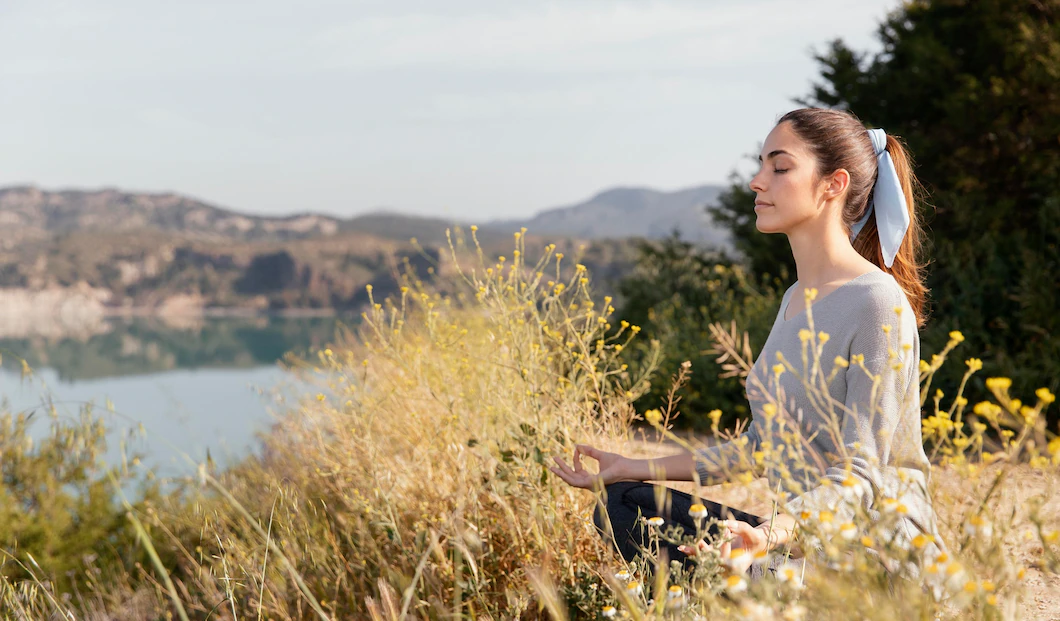# Healthy Minds, Healthy Workplaces

Challenges, adversities, and stressors at work are inevitable. According to statistics, employees have been more frustrated, burnout, and exhausted at work because of overwhelming and emotional stressors.

Do not journey alone as a healthy work-life balance is essential for peak performance. Here at Aventis Wellness, we prioritize your company and your employees’ mental well-being. Let us help you now!

```!function(t,n){"object"==typeof exports&&"undefined"!=typeof module?module.exports=n():"function"==typeof define&&define.amd?define(n):(t="undefined"!=typeof globalThis?globalThis:t||self).__SVGATOR_PLAYER__=n()}(this,(function(){"use strict";function t(n){return(t="function"==typeof Symbol&&"symbol"==typeof Symbol.iterator?function(t){return typeof t}:function(t){return t&&"function"==typeof Symbol&&t.constructor===Symbol&&t!==Symbol.prototype?"symbol":typeof t})(n)}function n(t,n){if(!(t instanceof n))throw new TypeError("Cannot call a class as a function")}function r(t,n){for(var r=0;r<n.length;r++){var e=n[r];e.enumerable=e.enumerable||!1,e.configurable=!0,"value"in e&&(e.writable=!0),Object.defineProperty(t,e.key,e)}}function e(t,n,e){return n&&r(t.prototype,n),e&&r(t,e),t}function i(t){return(i=Object.setPrototypeOf?Object.getPrototypeOf:function(t){return t.__proto__||Object.getPrototypeOf(t)})(t)}function o(t,n){return(o=Object.setPrototypeOf||function(t,n){return t.__proto__=n,t})(t,n)}function u(t,n){return!n||"object"!=typeof n&&"function"!=typeof n?function(t){if(void 0===t)throw new ReferenceError("this hasn't been initialised - super() hasn't been called");return t}(t):n}function a(t){var n=function(){if("undefined"==typeof Reflect||!Reflect.construct)return!1;if(Reflect.construct.sham)return!1;if("function"==typeof Proxy)return!0;try{return Boolean.prototype.valueOf.call(Reflect.construct(Boolean,[],(function(){}))),!0}catch(t){return!1}}();return function(){var r,e=i(t);if(n){var o=i(this).constructor;r=Reflect.construct(e,arguments,o)}else r=e.apply(this,arguments);return u(this,r)}}function l(t,n,r){return(l="undefined"!=typeof Reflect&&Reflect.get?Reflect.get:function(t,n,r){var e=function(t,n){for(;!Object.prototype.hasOwnProperty.call(t,n)&&null!==(t=i(t)););return t}(t,n);if(e){var o=Object.getOwnPropertyDescriptor(e,n);return o.get?o.get.call(r):o.value}})(t,n,r||t)}var f=s(Math.pow(10,-6));function s(t){var n=arguments.length>1&&void 0!==arguments?arguments:6;if(Number.isInteger(t))return t;var r=Math.pow(10,n);return Math.round((+t+Number.EPSILON)*r)/r}function c(t,n){var r=arguments.length>2&&void 0!==arguments?arguments:f;return Math.abs(t-n)<r}var h=Math.PI/180;function v(t){return t}function y(t,n,r){var e=1-r;return 3*r*e*(t*e+n*r)+r*r*r}function d(){var t=arguments.length>0&&void 0!==arguments?arguments:0,n=arguments.length>1&&void 0!==arguments?arguments:0,r=arguments.length>2&&void 0!==arguments?arguments:1,e=arguments.length>3&&void 0!==arguments?arguments:1;return t<0||t>1||r<0||r>1?null:c(t,n)&&c(r,e)?v:function(i){if(i<=0)return t>0?i*n/t:0===n&&r>0?i*e/r:0;if(i>=1)return r<1?1+(i-1)*(e-1)/(r-1):1===r&&t<1?1+(i-1)*(n-1)/(t-1):1;for(var o,u=0,a=1;u<a;){var l=y(t,r,o=(u+a)/2);if(c(i,l))break;l<i?u=o:a=o}return y(n,e,o)}}function g(){return 1}function p(t){return 1===t?1:0}function m(){var t=arguments.length>0&&void 0!==arguments?arguments:1,n=arguments.length>1&&void 0!==arguments?arguments:0;if(1===t){if(0===n)return p;if(1===n)return g}var r=1/t;return function(t){return t>=1?1:(t+=n*r)-t%r}}function b(t,n,r){return t>=.5?r:n}function w(t,n,r){return 0===t||n===r?n:t*(r-n)+n}function x(t,n,r){var e=w(t,n,r);return e<=0?0:e}function k(t,n,r){return 0===t?n:1===t?r:{x:w(t,n.x,r.x),y:w(t,n.y,r.y)}}function A(t,n,r){var e=function(t,n,r){return Math.round(w(t,n,r))}(t,n,r);return e<=0?0:e>=255?255:e}function S(t,n,r){return 0===t?n:1===t?r:{r:A(t,n.r,r.r),g:A(t,n.g,r.g),b:A(t,n.b,r.b),a:w(t,null==n.a?1:n.a,null==r.a?1:r.a)}}function _(t,n,r){if(0===t)return n;if(1===t)return r;var e=n.length;if(e!==r.length)return b(t,n,r);for(var i=[],o=0;o<e;o++)i.push(S(t,n[o],r[o]));return i}function O(t,n){for(var r=[],e=0;e<t;e++)r.push(n);return r}function M(t,n){if(--n<=0)return t;var r=(t=Object.assign([],t)).length;do{for(var e=0;e<r;e++)t.push(t[e])}while(--n>0);return t}var j,P=function(){function t(r){n(this,t),this.list=r,this.length=r.length}return e(t,[{key:"setAttribute",value:function(t,n){for(var r=this.list,e=0;e<this.length;e++)r[e].setAttribute(t,n)}},{key:"removeAttribute",value:function(t){for(var n=this.list,r=0;r<this.length;r++)n[r].removeAttribute(t)}},{key:"style",value:function(t,n){for(var r=this.list,e=0;e<this.length;e++)r[e].style[t]=n}}]),t}(),I=/-./g,E=function(t,n){return n.toUpperCase()};function F(t){return"function"==typeof t?t:b}function N(t){return t?"function"==typeof t?t:Array.isArray(t)?function(t){var n=arguments.length>1&&void 0!==arguments?arguments:v;if(!Array.isArray(t))return n;switch(t.length){case 1:return m(t)||n;case 2:return m(t,t)||n;case 4:return d(t,t,t,t)||n}return n}(t,null):function(t,n){var r=arguments.length>2&&void 0!==arguments?arguments:v;switch(t){case"linear":return v;case"steps":return m(n.steps||1,n.jump||0)||r;case"bezier":case"cubic-bezier":return d(n.x1||0,n.y1||0,n.x2||0,n.y2||0)||r}return r}(t.type,t.value,null):null}function B(t,n,r){var e=arguments.length>3&&void 0!==arguments&&arguments,i=n.length-1;if(t<=n.t)return e?[0,0,n.v]:n.v;if(t>=n[i].t)return e?[i,1,n[i].v]:n[i].v;var o,u=n,a=null;for(o=1;o<=i;o++){if(!(t>n[o].t)){a=n[o];break}u=n[o]}return null==a?e?[i,1,n[i].v]:n[i].v:u.t===a.t?e?[o,1,a.v]:a.v:(t=(t-u.t)/(a.t-u.t),u.e&&(t=u.e(t)),e?[o,t,r(t,u.v,a.v)]:r(t,u.v,a.v))}function R(t,n){var r=arguments.length>2&&void 0!==arguments?arguments:null;return t&&t.length?"function"!=typeof n?null:("function"!=typeof r&&(r=null),function(e){var i=B(e,t,n);return null!=i&&r&&(i=r(i)),i}):null}function q(t,n){return t.t-n.t}function T(n,r,e,i,o){var u,a="@"===e,l="#"===e,f=j[e],s=b;switch(a?(u=e.substr(1),e=u.replace(I,E)):l&&(e=e.substr(1)),t(f)){case"function":if(s=f(i,o,B,N,e,a,r,n),l)return s;break;case"string":s=R(i,F(f));break;case"object":if((s=R(i,F(f.i),f.f))&&"function"==typeof f.u)return f.u(r,s,e,a,n)}return s?function(t,n,r){var e=arguments.length>3&&void 0!==arguments&&arguments;if(e)return t instanceof P?function(e){return t.style(n,r(e))}:function(e){return t.style[n]=r(e)};if(Array.isArray(n)){var i=n.length;return function(e){var o=r(e);if(null==o)for(var u=0;u<i;u++)t[u].removeAttribute(n);else for(var a=0;a<i;a++)t[a].setAttribute(n,o)}}return function(e){var i=r(e);null==i?t.removeAttribute(n):t.setAttribute(n,i)}}(r,e,s,a):null}function L(n,r,e,i){if(!i||"object"!==t(i))return null;var o=null,u=null;return Array.isArray(i)?u=function(t){if(!t||!t.length)return null;for(var n=0;n<t.length;n++)t[n].e&&(t[n].e=N(t[n].e));return t.sort(q)}(i):(u=i.keys,o=i.data||null),u?T(n,r,e,u,o):null}function C(t,n,r){if(!r)return null;var e=[];for(var i in r)if(r.hasOwnProperty(i)){var o=L(t,n,i,r[i]);o&&e.push(o)}return e.length?e:null}function z(t,n){if(!n.duration||n.duration<0)return null;var r=function(t,n){if(!n)return null;var r=[];if(Array.isArray(n))for(var e=n.length,i=0;i<e;i++){var o=n[i];if(2===o.length){var u=null;if("string"==typeof o)u=t.getElementById(o);else if(Array.isArray(o)){u=[];for(var a=0;a<o.length;a++)if("string"==typeof o[a]){var l=t.getElementById(o[a]);l&&u.push(l)}u=u.length?1===u.length?u:new P(u):null}if(u){var f=C(t,u,o);f&&(r=r.concat(f))}}}else for(var s in n)if(n.hasOwnProperty(s)){var c=t.getElementById(s);if(c){var h=C(t,c,n[s]);h&&(r=r.concat(h))}}return r.length?r:null}(t,n.elements);return r?function(t,n){var r=arguments.length>2&&void 0!==arguments?arguments:1/0,e=arguments.length>3&&void 0!==arguments?arguments:1,i=arguments.length>4&&void 0!==arguments&&arguments,o=arguments.length>5&&void 0!==arguments?arguments:1,u=t.length,a=e>0?n:0;i&&r%2==0&&(a=n-a);var l=null;return function(f,s){var c=f%n,h=1+(f-c)/n;s*=e,i&&h%2==0&&(s=-s);var v=!1;if(h>r)c=a,v=!0,-1===o&&(c=e>0?0:n);else if(s<0&&(c=n-c),c===l)return!1;l=c;for(var y=0;y<u;y++)t[y](c);return v}}(r,n.duration,n.iterations||1/0,n.direction||1,!!n.alternate,n.fill||1):null}Number.isInteger||(Number.isInteger=function(t){return"number"==typeof t&&isFinite(t)&&Math.floor(t)===t}),Number.EPSILON||(Number.EPSILON=2220446049250313e-31);var D=function(){function t(r,e){var i=arguments.length>2&&void 0!==arguments?arguments:{};n(this,t),this._id=0,this._running=!1,this._rollingBack=!1,this._animations=r,this.duration=e.duration,this.alternate=e.alternate,this.fill=e.fill,this.iterations=e.iterations,this.direction=i.direction||1,this.speed=i.speed||1,this.fps=i.fps||100,this.offset=i.offset||0,this.rollbackStartOffset=0}return e(t,[{key:"_rollback",value:function(){var t=this,n=1/0,r=null;this.rollbackStartOffset=this.offset,this._rollingBack||(this._rollingBack=!0,this._running=!0);this._id=window.requestAnimationFrame((function e(i){if(t._rollingBack){null==r&&(r=i);var o=i-r,u=t.rollbackStartOffset-o,a=Math.round(u*t.speed);if(a>t.duration&&n!=1/0){var l=!!t.alternate&&a/t.duration%2>1,f=a%t.duration;a=(f+=l?t.duration:0)||t.duration}var s=t.fps?1e3/t.fps:0,c=Math.max(0,a);if(c<n-s){t.offset=c,n=c;for(var h=t._animations,v=h.length,y=0;y<v;y++)h[y](c,t.direction)}var d=!1;if(t.iterations>0&&-1===t.fill){var g=t.iterations*t.duration,p=g==a;a=p?0:a,t.offset=p?0:t.offset,d=a>g}a>0&&t.offset>=a&&!d?t._id=window.requestAnimationFrame(e):t.stop()}}))}},{key:"_start",value:function(){var t=this,n=arguments.length>0&&void 0!==arguments?arguments:0,r=-1/0,e=null,i={},o=function o(u){t._running=!0,null==e&&(e=u);var a=Math.round((u-e+n)*t.speed),l=t.fps?1e3/t.fps:0;if(a>r+l&&!t._rollingBack){t.offset=a,r=a;for(var f=t._animations,s=f.length,c=0,h=0;h<s;h++)i[h]?c++:(i[h]=f[h](a,t.direction),i[h]&&c++);if(c===s)return void t._stop()}t._id=window.requestAnimationFrame(o)};this._id=window.requestAnimationFrame(o)}},{key:"_stop",value:function(){this._id&&window.cancelAnimationFrame(this._id),this._running=!1,this._rollingBack=!1}},{key:"play",value:function(){!this._rollingBack&&this._running||(this._rollingBack=!1,this.rollbackStartOffset>this.duration&&(this.offset=this.rollbackStartOffset-(this.rollbackStartOffset-this.offset)%this.duration,this.rollbackStartOffset=0),this._start(this.offset))}},{key:"stop",value:function(){this._stop(),this.offset=0,this.rollbackStartOffset=0;var t=this.direction,n=this._animations;window.requestAnimationFrame((function(){for(var r=0;r<n.length;r++)n[r](0,t)}))}},{key:"reachedToEnd",value:function(){return this.iterations>0&&this.offset>=this.iterations*this.duration}},{key:"restart",value:function(){this._stop(),this.offset=0,this._start()}},{key:"pause",value:function(){this._stop()}},{key:"reverse",value:function(){this.direction=-this.direction}}],[{key:"build",value:function(n,r){return(n=function(t,n){if(j=n,!t||!t.root||!Array.isArray(t.animations))return null;for(var r=document.getElementsByTagName("svg"),e=!1,i=0;i<r.length;i++)if(r[i].id===t.root&&!r[i].svgatorAnimation){(e=r[i]).svgatorAnimation=!0;break}if(!e)return null;var o=t.animations.map((function(t){return z(e,t)})).filter((function(t){return!!t}));return o.length?{element:e,animations:o,animationSettings:t.animationSettings,options:t.options||void 0}:null}(n,r))?{el:n.element,options:n.options||{},player:new t(n.animations,n.animationSettings,n.options)}:null}}]),t}();function Q(t){return s(t)+""}function U(t){var n=arguments.length>1&&void 0!==arguments?arguments:" ";return t&&t.length?t.map(Q).join(n):""}function V(t){return t?null==t.a||t.a>=1?"rgb("+t.r+","+t.g+","+t.b+")":"rgba("+t.r+","+t.g+","+t.b+","+t.a+")":"transparent"}!function(){for(var t=0,n=["ms","moz","webkit","o"],r=0;r<n.length&&!window.requestAnimationFrame;++r)window.requestAnimationFrame=window[n[r]+"RequestAnimationFrame"],window.cancelAnimationFrame=window[n[r]+"CancelAnimationFrame"]||window[n[r]+"CancelRequestAnimationFrame"];window.requestAnimationFrame||(window.requestAnimationFrame=function(n){var r=Date.now(),e=Math.max(0,16-(r-t)),i=window.setTimeout((function(){n(r+e)}),e);return t=r+e,i},window.cancelAnimationFrame=window.clearTimeout)}();var G={f:null,i:function(t,n,r){return 0===t?n:1===t?r:{x:x(t,n.x,r.x),y:x(t,n.y,r.y)}},u:function(t,n){return function(r){var e=n(r);t.setAttribute("rx",Q(e.x)),t.setAttribute("ry",Q(e.y))}}},H={f:null,i:function(t,n,r){return 0===t?n:1===t?r:{width:x(t,n.width,r.width),height:x(t,n.height,r.height)}},u:function(t,n){return function(r){var e=n(r);t.setAttribute("width",Q(e.width)),t.setAttribute("height",Q(e.height))}}},Y=Math.sin,Z=Math.cos,J=Math.acos,K=Math.asin,W=Math.tan,X=Math.atan2,\$=Math.PI/180,tt=180/Math.PI,nt=Math.sqrt,rt=function(){function t(){var r=arguments.length>0&&void 0!==arguments?arguments:1,e=arguments.length>1&&void 0!==arguments?arguments:0,i=arguments.length>2&&void 0!==arguments?arguments:0,o=arguments.length>3&&void 0!==arguments?arguments:1,u=arguments.length>4&&void 0!==arguments?arguments:0,a=arguments.length>5&&void 0!==arguments?arguments:0;n(this,t),this.m=[r,e,i,o,u,a],this.i=null,this.w=null,this.s=null}return e(t,[{key:"determinant",get:function(){var t=this.m;return t*t-t*t}},{key:"isIdentity",get:function(){if(null===this.i){var t=this.m;this.i=1===t&&0===t&&0===t&&1===t&&0===t&&0===t}return this.i}},{key:"point",value:function(t,n){var r=this.m;return{x:r*t+r*n+r,y:r*t+r*n+r}}},{key:"translateSelf",value:function(){var t=arguments.length>0&&void 0!==arguments?arguments:0,n=arguments.length>1&&void 0!==arguments?arguments:0;if(!t&&!n)return this;var r=this.m;return r+=r*t+r*n,r+=r*t+r*n,this.w=this.s=this.i=null,this}},{key:"rotateSelf",value:function(){var t=arguments.length>0&&void 0!==arguments?arguments:0;if(t%=360){var n=Y(t*=\$),r=Z(t),e=this.m,i=e,o=e;e=i*r+e*n,e=o*r+e*n,e=e*r-i*n,e=e*r-o*n,this.w=this.s=this.i=null}return this}},{key:"scaleSelf",value:function(){var t=arguments.length>0&&void 0!==arguments?arguments:1,n=arguments.length>1&&void 0!==arguments?arguments:1;if(1!==t||1!==n){var r=this.m;r*=t,r*=t,r*=n,r*=n,this.w=this.s=this.i=null}return this}},{key:"skewSelf",value:function(t,n){if(n%=360,(t%=360)||n){var r=this.m,e=r,i=r,o=r,u=r;t&&(t=W(t*\$),r+=e*t,r+=i*t),n&&(n=W(n*\$),r+=o*n,r+=u*n),this.w=this.s=this.i=null}return this}},{key:"resetSelf",value:function(){var t=arguments.length>0&&void 0!==arguments?arguments:1,n=arguments.length>1&&void 0!==arguments?arguments:0,r=arguments.length>2&&void 0!==arguments?arguments:0,e=arguments.length>3&&void 0!==arguments?arguments:1,i=arguments.length>4&&void 0!==arguments?arguments:0,o=arguments.length>5&&void 0!==arguments?arguments:0,u=this.m;return u=t,u=n,u=r,u=e,u=i,u=o,this.w=this.s=this.i=null,this}},{key:"recomposeSelf",value:function(){var t=arguments.length>0&&void 0!==arguments?arguments:null,n=arguments.length>1&&void 0!==arguments?arguments:null,r=arguments.length>2&&void 0!==arguments?arguments:null,e=arguments.length>3&&void 0!==arguments?arguments:null,i=arguments.length>4&&void 0!==arguments?arguments:null;return this.isIdentity||this.resetSelf(),t&&(t.x||t.y)&&this.translateSelf(t.x,t.y),n&&this.rotateSelf(n),r&&(r.x&&this.skewSelf(r.x,0),r.y&&this.skewSelf(0,r.y)),!e||1===e.x&&1===e.y||this.scaleSelf(e.x,e.y),i&&(i.x||i.y)&&this.translateSelf(i.x,i.y),this}},{key:"decompose",value:function(){var t=arguments.length>0&&void 0!==arguments?arguments:0,n=arguments.length>1&&void 0!==arguments?arguments:0,r=this.m,e=r*r+r*r,i=[[r,r],[r,r]],o=nt(e);if(0===o)return{origin:{x:s(r),y:s(r)},translate:{x:s(t),y:s(n)},scale:{x:0,y:0},skew:{x:0,y:0},rotate:0};i/=o,i/=o;var u=r*r-r*r<0;u&&(o=-o);var a=i*i+i*i;i-=i*a,i-=i*a;var l=nt(i*i+i*i);if(0===l)return{origin:{x:s(r),y:s(r)},translate:{x:s(t),y:s(n)},scale:{x:s(o),y:0},skew:{x:0,y:0},rotate:0};i/=l,i/=l,a/=l;var f=0;return i<0?(f=J(i)*tt,i<0&&(f=360-f)):f=K(i)*tt,u&&(f=-f),a=X(a,nt(i*i+i*i))*tt,u&&(a=-a),{origin:{x:s(r),y:s(r)},translate:{x:s(t),y:s(n)},scale:{x:s(o),y:s(l)},skew:{x:s(a),y:0},rotate:s(f)}}},{key:"toString",value:function(){return null===this.s&&(this.s="matrix("+this.m.map((function(t){return s(t)})).join(" ")+")"),this.s}}]),t}();Object.freeze({M:2,L:2,Z:0,H:1,V:1,C:6,Q:4,T:2,S:4,A:7});var et={},it=null;function ot(n){var r=function(){if(it)return it;if("object"!==("undefined"==typeof document?"undefined":t(document))||!document.createElementNS)return{};var n=document.createElementNS("http://www.w3.org/2000/svg","svg");return n&&n.style?(n.style.position="absolute",n.style.opacity="0.01",n.style.zIndex="-9999",n.style.left="-9999px",n.style.width="1px",n.style.height="1px",it={svg:n}):{}}().svg;if(!r)return function(t){return null};var e=document.createElementNS(r.namespaceURI,"path");e.setAttributeNS(null,"d",n),e.setAttributeNS(null,"fill","none"),e.setAttributeNS(null,"stroke","none"),r.appendChild(e);var i=e.getTotalLength();return function(t){var n=e.getPointAtLength(i*t);return{x:n.x,y:n.y}}}function ut(t){return et[t]?et[t]:et[t]=ot(t)}function at(t,n,r,e){if(!t||!e)return!1;var i=["M",t.x,t.y];if(n&&r&&(i.push("C"),i.push(n.x),i.push(n.y),i.push(r.x),i.push(r.y)),n?!r:r){var o=n||r;i.push("Q"),i.push(o.x),i.push(o.y)}return n||r||i.push("L"),i.push(e.x),i.push(e.y),i.join(" ")}function lt(t,n,r,e){var i=arguments.length>4&&void 0!==arguments?arguments:1,o=at(t,n,r,e),u=ut(o);try{return u(i)}catch(t){return null}}function ft(t,n,r,e){var i=1-e;return i*i*t+2*i*e*n+e*e*r}function st(t,n,r,e){return 2*(1-e)*(n-t)+2*e*(r-n)}function ct(t,n,r,e){var i=arguments.length>4&&void 0!==arguments&&arguments,o=lt(t,n,null,r,e);return o||(o={x:ft(t.x,n.x,r.x,e),y:ft(t.y,n.y,r.y,e)}),i&&(o.a=ht(t,n,r,e)),o}function ht(t,n,r,e){return Math.atan2(st(t.y,n.y,r.y,e),st(t.x,n.x,r.x,e))}function vt(t,n,r,e,i){var o=i*i;return i*o*(e-t+3*(n-r))+3*o*(t+r-2*n)+3*i*(n-t)+t}function yt(t,n,r,e,i){var o=1-i;return 3*(o*o*(n-t)+2*o*i*(r-n)+i*i*(e-r))}function dt(t,n,r,e,i){var o=arguments.length>5&&void 0!==arguments&&arguments,u=lt(t,n,r,e,i);return u||(u={x:vt(t.x,n.x,r.x,e.x,i),y:vt(t.y,n.y,r.y,e.y,i)}),o&&(u.a=gt(t,n,r,e,i)),u}function gt(t,n,r,e,i){return Math.atan2(yt(t.y,n.y,r.y,e.y,i),yt(t.x,n.x,r.x,e.x,i))}function pt(t,n,r){return t+(n-t)*r}function mt(t,n,r){var e=arguments.length>3&&void 0!==arguments&&arguments,i={x:pt(t.x,n.x,r),y:pt(t.y,n.y,r)};return e&&(i.a=bt(t,n)),i}function bt(t,n){return Math.atan2(n.y-t.y,n.x-t.x)}function wt(t,n,r){var e=arguments.length>3&&void 0!==arguments&&arguments;if(kt(n)){if(At(r))return ct(n,r.start,r,t,e)}else if(kt(r)){if(n.end)return ct(n,n.end,r,t,e)}else{if(n.end)return r.start?dt(n,n.end,r.start,r,t,e):ct(n,n.end,r,t,e);if(r.start)return ct(n,r.start,r,t,e)}return mt(n,r,t,e)}function xt(t,n,r){var e=wt(t,n,r,!0);return e.a=function(t){var n=arguments.length>1&&void 0!==arguments&&arguments;return n?t+Math.PI:t}(e.a)/h,e}function kt(t){return!t.type||"corner"===t.type}function At(t){return null!=t.start&&!kt(t)}var St=new rt;var _t={f:Q,i:w},Ot={f:Q,i:function(t,n,r){var e=w(t,n,r);return e<=0?0:e>=1?1:e}};function Mt(t,n,r,e,i,o,u,a){return n=function(t,n,r){for(var e,i,o,u=t.length-1,a={},l=0;l<=u;l++)(e=t[l]).e&&(e.e=n(e.e)),e.v&&"g"===(i=e.v).t&&i.r&&(o=r.getElementById(i.r))&&(a[i.r]=o.querySelectorAll("stop"));return a}(t,e,a),function(e){var i,o=r(e,t,jt);return o?"c"===o.t?V(o.v):"g"===o.t?(n[o.r]&&function(t,n){for(var r=0,e=t.length;r<e;r++)t[r].setAttribute("stop-color",V(n[r]))}(n[o.r],o.v),(i=o.r)?"url(#"+i+")":"none"):"none":"none"}}function jt(t,n,r){if(0===t)return n;if(1===t)return r;if(n&&r){var e=n.t;if(e===r.t)switch(n.t){case"c":return{t:e,v:S(t,n.v,r.v)};case"g":if(n.r===r.r)return{t:e,v:_(t,n.v,r.v),r:n.r}}}return b(t,n,r)}var Pt={fill:Mt,"fill-opacity":Ot,stroke:Mt,"stroke-opacity":Ot,"stroke-width":_t,"stroke-dashoffset":{f:Q,i:w},"stroke-dasharray":{f:function(t){var n=arguments.length>1&&void 0!==arguments?arguments:" ";return t&&t.length>0&&(t=t.map((function(t){return s(t,4)}))),U(t,n)},i:function(t,n,r){var e,i,o,u=n.length,a=r.length;if(u!==a)if(0===u)n=O(u=a,0);else if(0===a)a=u,r=O(u,0);else{var l=(o=(e=u)*(i=a)/function(t,n){for(var r;n;)r=n,n=t%n,t=r;return t||1}(e,i))<0?-o:o;n=M(n,Math.floor(l/u)),r=M(r,Math.floor(l/a)),u=a=l}for(var f=[],c=0;c<u;c++)f.push(s(x(t,n[c],r[c])));return f}},opacity:Ot,transform:function(n,r,e,i){if(!(n=function(n,r){if(!n||"object"!==t(n))return null;var e=!1;for(var i in n)n.hasOwnProperty(i)&&(n[i]&&n[i].length?(n[i].forEach((function(t){t.e&&(t.e=r(t.e))})),e=!0):delete n[i]);return e?n:null}(n,i)))return null;var o=function(t,i,o){var u=arguments.length>3&&void 0!==arguments?arguments:null;return n[t]?e(i,n[t],o):r&&r[t]?r[t]:u};return r&&r.a&&n.o?function(t){var r=e(t,n.o,xt);return St.recomposeSelf(r,o("r",t,w,0)+r.a,o("k",t,k),o("s",t,k),o("t",t,k)).toString()}:function(t){return St.recomposeSelf(o("o",t,wt,null),o("r",t,w,0),o("k",t,k),o("s",t,k),o("t",t,k)).toString()}},r:_t,"#size":H,"#radius":G,_:function(t,n){if(Array.isArray(t))for(var r=0;r<t.length;r++)this[t[r]]=n;else this[t]=n}};return function(t){!function(t,n){if("function"!=typeof n&&null!==n)throw new TypeError("Super expression must either be null or a function");t.prototype=Object.create(n&&n.prototype,{constructor:{value:t,writable:!0,configurable:!0}}),n&&o(t,n)}(u,t);var r=a(u);function u(){return n(this,u),r.apply(this,arguments)}return e(u,null,[{key:"build",value:function(t){var n=l(i(u),"build",this).call(this,t,Pt);if(!n)return null;n.el,n.options;var r=n.player;return function(t,n,r){t.play()}(r),r}}]),u}(D)})); __SVGATOR_PLAYER__.build({"root":"ey5gncJ1jWK1","animations":[{"duration":3000,"direction":1,"iterations":0,"fill":1,"alternate":true,"speed":1,"elements":{"ey5gncJ1jWK10":{"transform":{"data":{"t":{"x":-573.0625,"y":-108.384766}},"keys":{"o":[{"t":70,"v":{"x":573.0625,"y":108.384766,"type":"corner"},"e":[0.42,0,0.58,1]},{"t":1150,"v":{"x":573.0625,"y":124.00793,"type":"corner"},"e":[0.42,0,0.58,1]},{"t":2000,"v":{"x":573.0625,"y":106.285747,"type":"corner"}}]}}},"ey5gncJ1jWK18":{"transform":{"data":{"t":{"x":-490.609375,"y":-43.539062}},"keys":{"o":[{"t":60,"v":{"x":490.609375,"y":43.539063,"type":"corner"},"e":[0.42,0,0.58,1]},{"t":1150,"v":{"x":490.609375,"y":65.215462,"type":"corner"},"e":[0.42,0,0.58,1]},{"t":2000,"v":{"x":490.609375,"y":47.763262,"type":"corner"}}]}}},"ey5gncJ1jWK24":{"transform":{"data":{"t":{"x":-462.333984,"y":-126.205078}},"keys":{"o":[{"t":50,"v":{"x":462.333985,"y":126.205079,"type":"corner"},"e":[0.42,0,0.58,1]},{"t":1150,"v":{"x":462.333985,"y":142.229355,"type":"corner"},"e":[0.42,0,0.58,1]},{"t":1990,"v":{"x":462.333985,"y":119.077891,"type":"corner"}}]}}},"ey5gncJ1jWK32":{"transform":{"data":{"t":{"x":-397.841797,"y":-75.597656}},"keys":{"o":[{"t":40,"v":{"x":397.841797,"y":75.597657,"type":"corner"},"e":[0.42,0,0.58,1]},{"t":1150,"v":{"x":397.841797,"y":94.742643,"type":"corner"},"e":[0.42,0,0.58,1]},{"t":1990,"v":{"x":397.841797,"y":72.412288,"type":"corner"}}]}}},"ey5gncJ1jWK46":{"transform":{"data":{"t":{"x":-273.960937,"y":-114.800781}},"keys":{"o":[{"t":30,"v":{"x":273.960938,"y":114.800782,"type":"corner"},"e":[0.42,0,0.58,1]},{"t":1150,"v":{"x":273.960938,"y":132.192735,"type":"corner"},"e":[0.42,0,0.58,1]},{"t":1990,"v":{"x":273.960938,"y":109.090382,"type":"corner"}}]}}},"ey5gncJ1jWK49":{"transform":{"data":{"t":{"x":-308.949219,"y":-44.236328}},"keys":{"o":[{"t":20,"v":{"x":308.949219,"y":44.236329,"type":"corner"},"e":[0.42,0,0.58,1]},{"t":1150,"v":{"x":308.949219,"y":55.890549,"type":"corner"},"e":[0.42,0,0.58,1]},{"t":1990,"v":{"x":308.949219,"y":44.465301,"type":"corner"}}]}}},"ey5gncJ1jWK59":{"transform":{"data":{"t":{"x":-194.242187,"y":-119.175781}},"keys":{"o":[{"t":10,"v":{"x":194.242188,"y":119.175782,"type":"corner"},"e":[0.42,0,0.58,1]},{"t":1150,"v":{"x":194.24219,"y":132.706803,"type":"corner"},"e":[0.42,0,0.58,1]},{"t":1980,"v":{"x":194.24219,"y":118.532371,"type":"corner"}}]}}},"ey5gncJ1jWK64":{"transform":{"data":{"t":{"x":-143.478516,"y":-101.394531}},"keys":{"o":[{"t":0,"v":{"x":143.478516,"y":101.394532,"type":"corner"},"e":[0.42,0,0.58,1]},{"t":1150,"v":{"x":143.478516,"y":113.366188,"type":"corner"},"e":[0.42,0,0.58,1]},{"t":1980,"v":{"x":143.478516,"y":95.820914,"type":"corner"}}]}}},"ey5gncJ1jWK139":{"opacity":[{"t":2450,"v":0},{"t":2480,"v":1}]}}}],"options":{"start":"load","hover":null,"click":null,"scroll":null,"font":"embed","exportedIds":"unique","exportCanvasColor":true,"svgFormat":"animated","title":"Graphic 2.4"},"animationSettings":{"duration":3000,"direction":1,"iterations":0,"fill":1,"alternate":true,"speed":1}})```

# How It Works

At Aventis Wellness, we are here to help. With over a decade of experience, we provide convenient, affordable and effective counselling for individuals and companies seeking to support their employees' mental wellbeing in a safe, non-judgmental and comfortable environment.

### For Companies

Step 1
Fill up the EAP enquiry form under “Contact Us” or Whatsapp 90822433 directly.

Step 2
Discuss with us what wellness-related trainings your company is looking at and decide on the number of credits to grant your staff for the redemption of therapy sessions with our therapists.

Step 3
Seamless onboarding by confirming dates and quotations. Materials will be provided to your organization on how they can utilize our system.

### For Individuals

Step 1

Step 2

Step 3
Schedule an appointment with your personal therapist.

Step 4
Commit time and journey together with your AW Therapist to move towards your goal and achieve a therapeutic alliance with your therapist.

## Our Services and Expertise

We provide Bespoke Customized Employee Assistance Program (EAP) Solutions to organizations including the following services:

• Wellness Coaching
• Work-Life Support
• Workshops
• Workplace Counselling
• Mental Wellness Clinic
• Mindfulness Workshop
• Lunch Talks on Mental Well Being Topics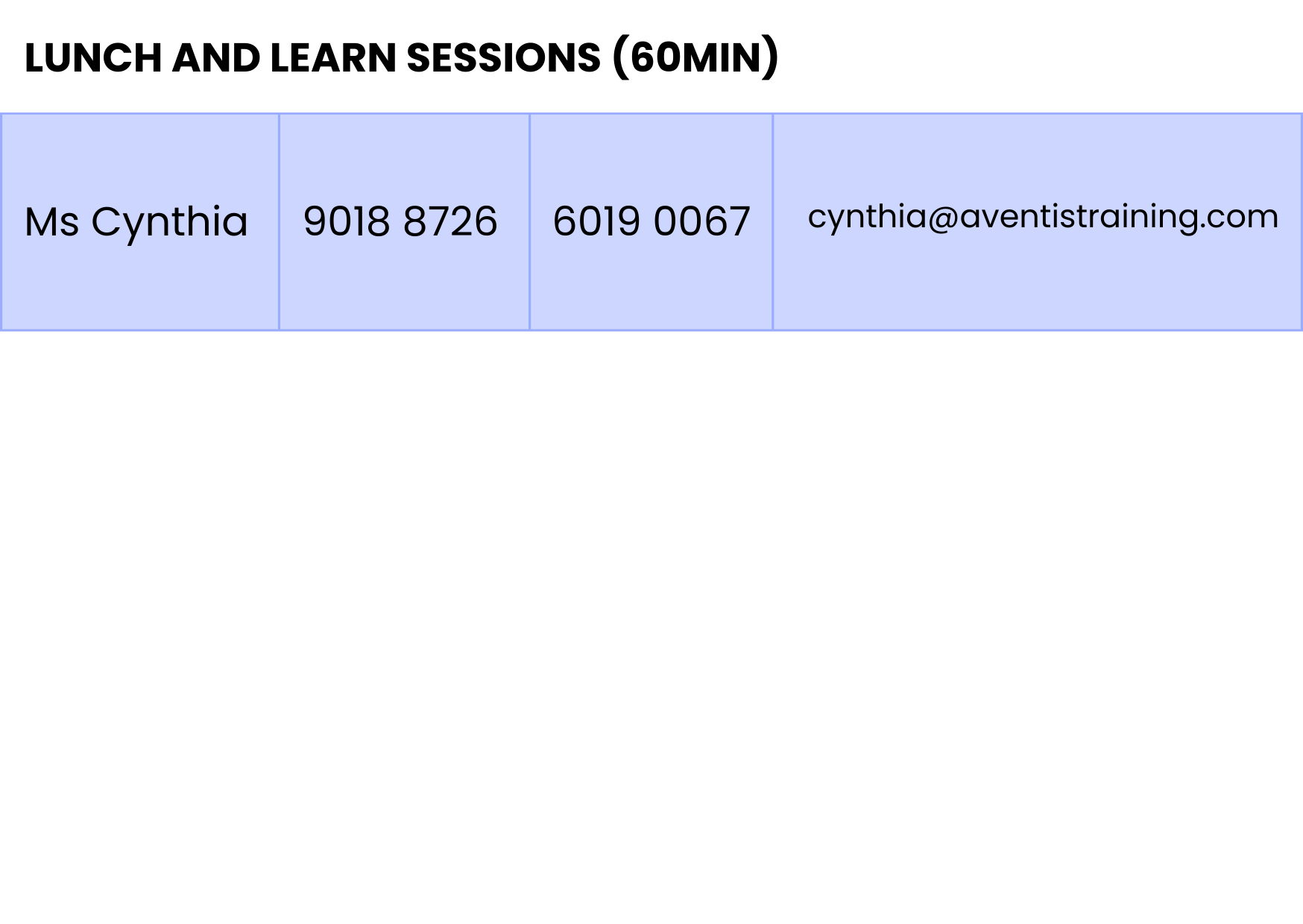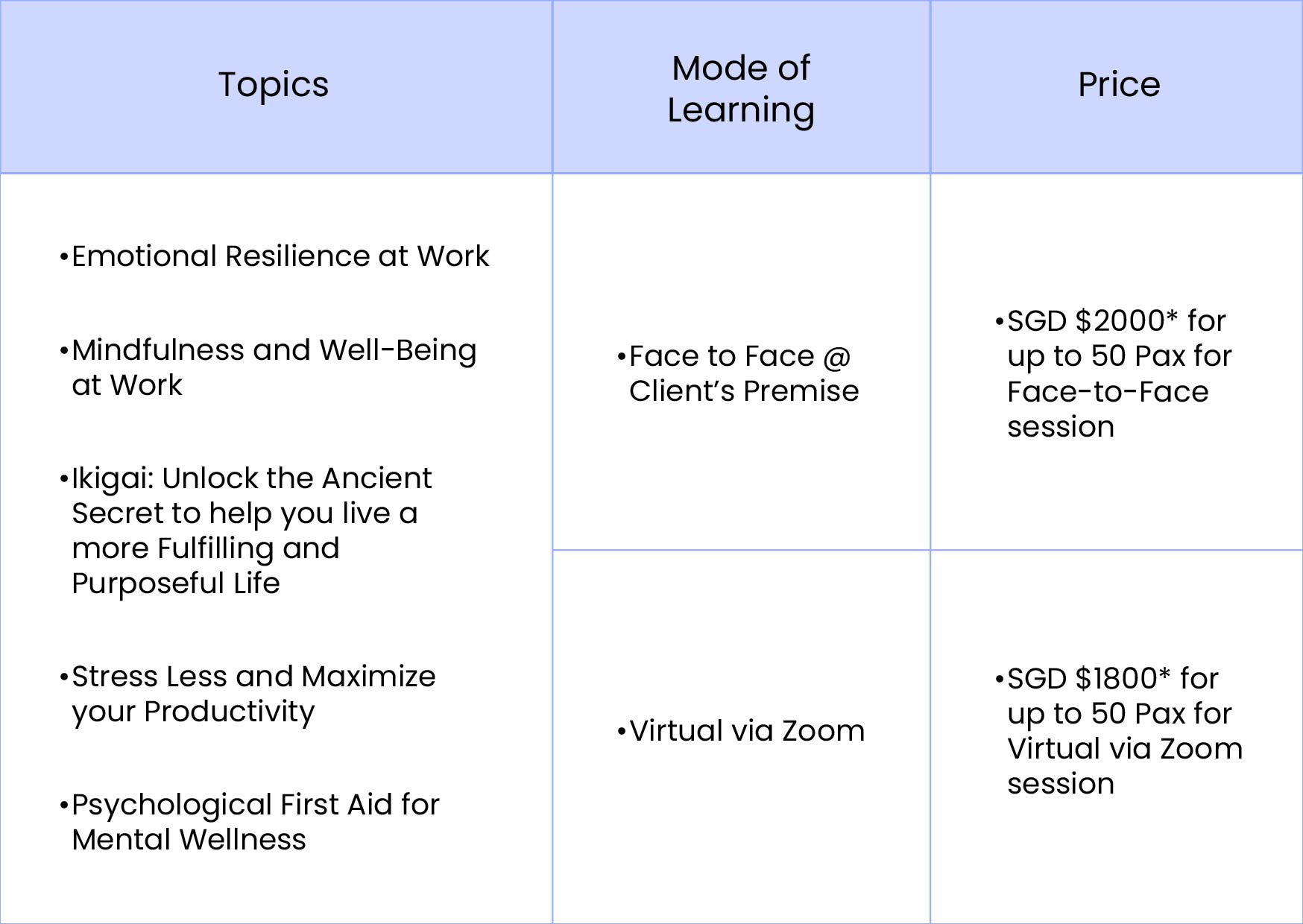## Our Clients & Partners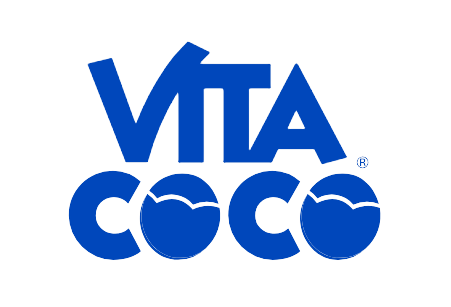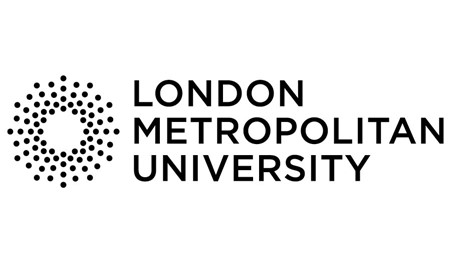## As featured On## What Others Have to Say

### Find out more about Aventis Wellness Employee Assistance Programme (EAP)

Thank you and have a lovely day ahead.

##### Participate now!

Mindfulness and Wellbeing

Speaker:  Erin Lee (Founder and Mindfulness Coach of Mindful Moments Singapore)

Date: 28th April 2023 (Friday)
Time: 4 PM-5 PM